AlgorithmsDijkstraGlossary

# Dijkstra’s Algorithm

#### Dijkstra’s algorithm allows us to find the shortest path between any two vertices of a graph.

It differs from the minimum spanning tree because the shortest distance between two vertices might not include all the vertices of the graph.

## How Dijkstra’s Algorithm works

Dijkstra’s Algorithm works on the basis that any subpath `B -> D` of the shortest path `A -> D` between vertices A and D is also the shortest path between vertices B and D.Each subpath is the shortest path

Djikstra used this property in the opposite direction i.e we overestimate the distance of each vertex from the starting vertex. Then we visit each node and its neighbors to find the shortest subpath to those neighbors.

The algorithm uses a greedy approach in the sense that we find the next best solution hoping that the end result is the best solution for the whole problem.

## Example of Dijkstra’s algorithm

It is easier to start with an example and then think about the algorithm.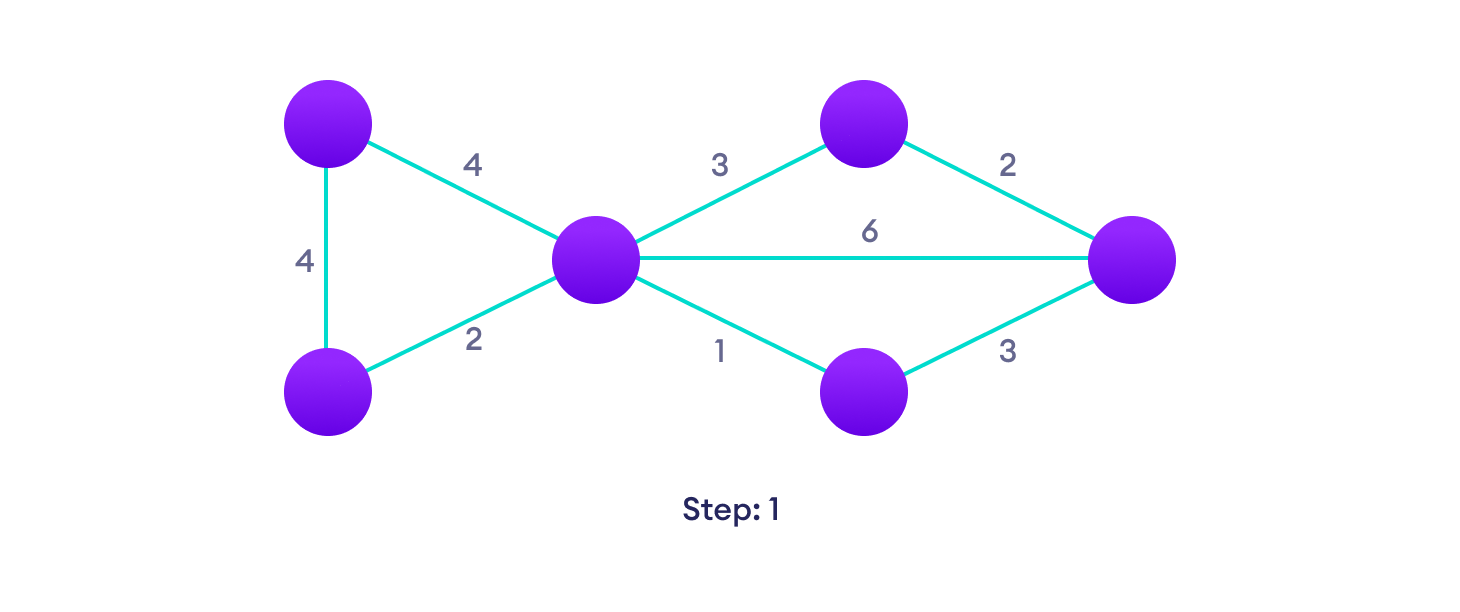Start with a weighted graph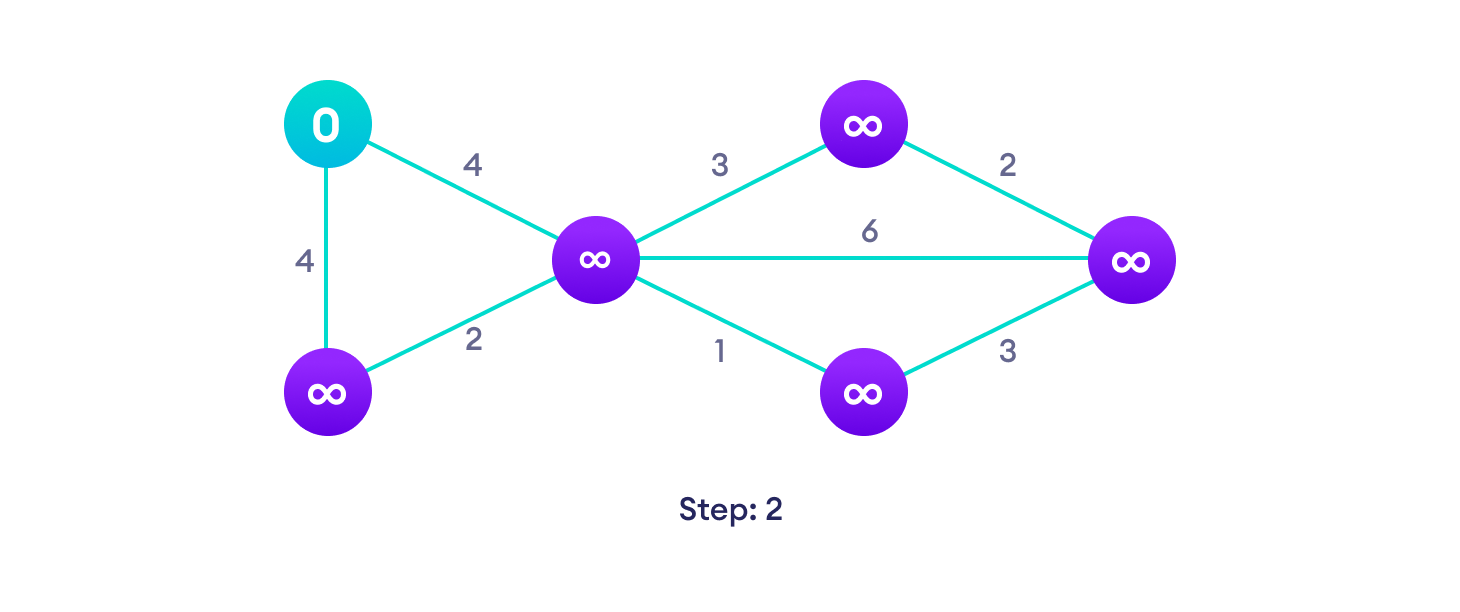Choose a starting vertex and assign infinity path values to all other devices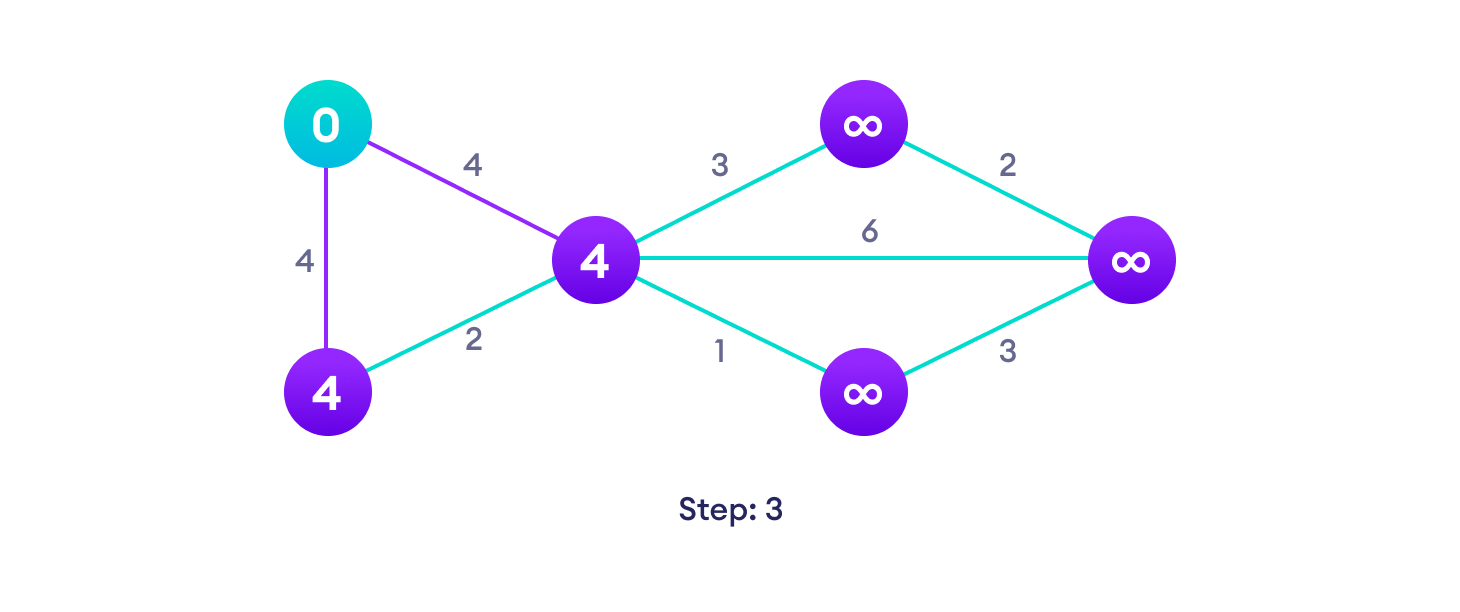Go to each vertex and update its path length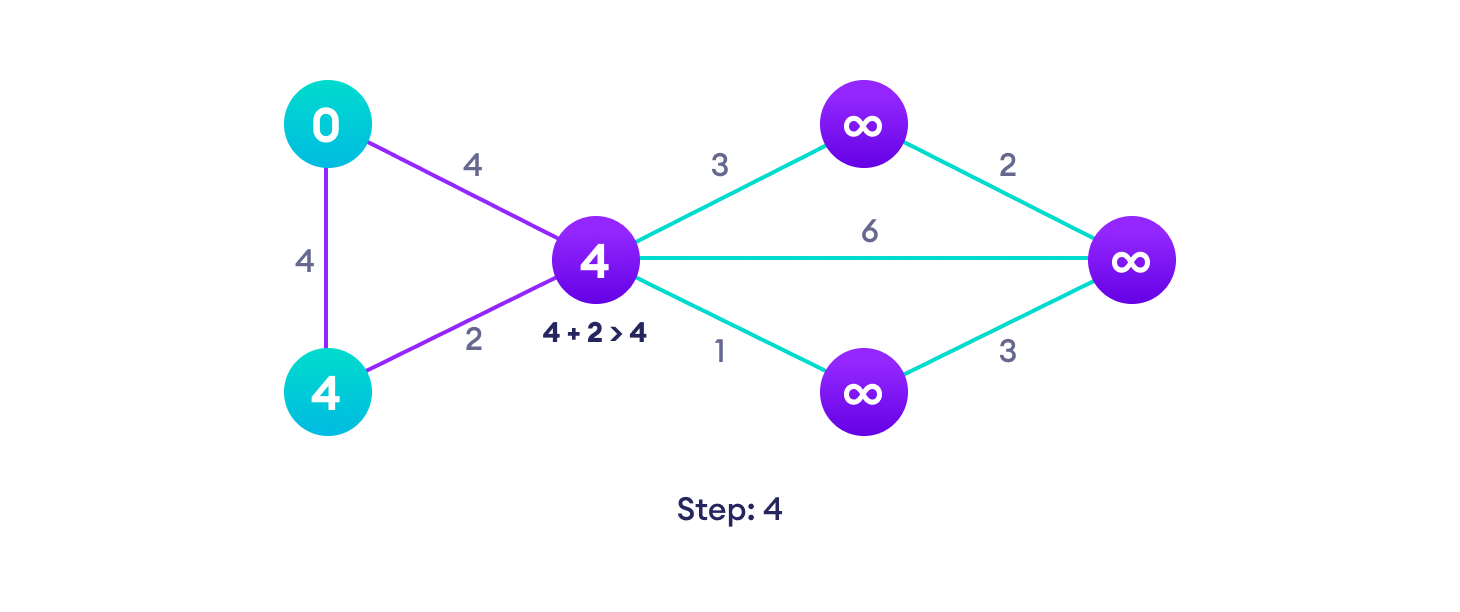If the path length of the adjacent vertex is lesser than new path length, don’t update it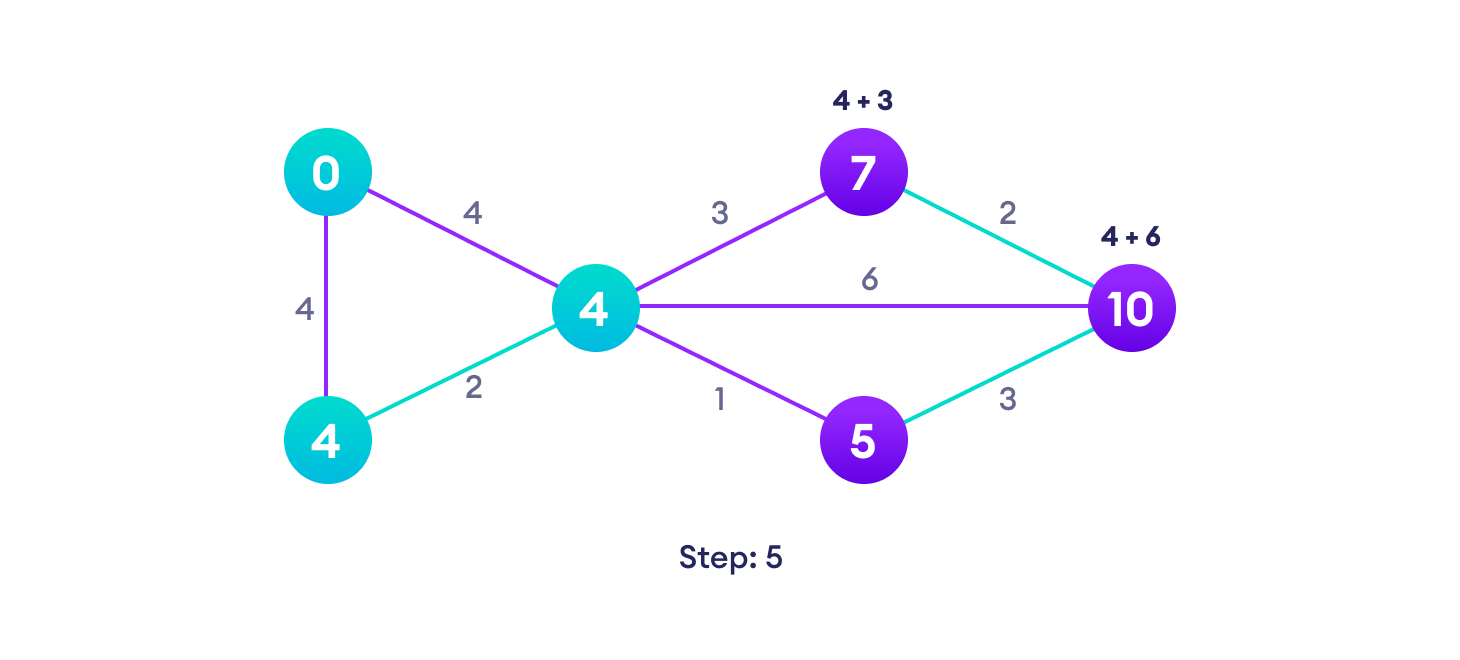Avoid updating path lengths of already visited vertices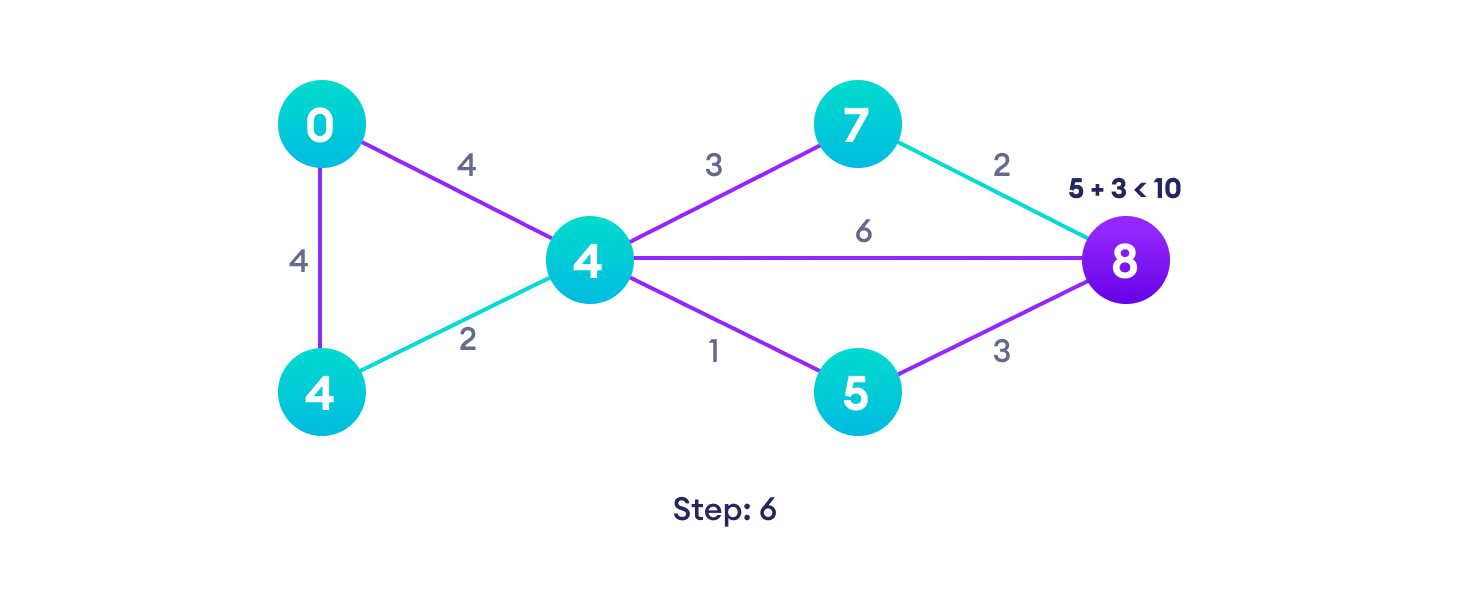After each iteration, we pick the unvisited vertex with the least path length. So we choose 5 before 7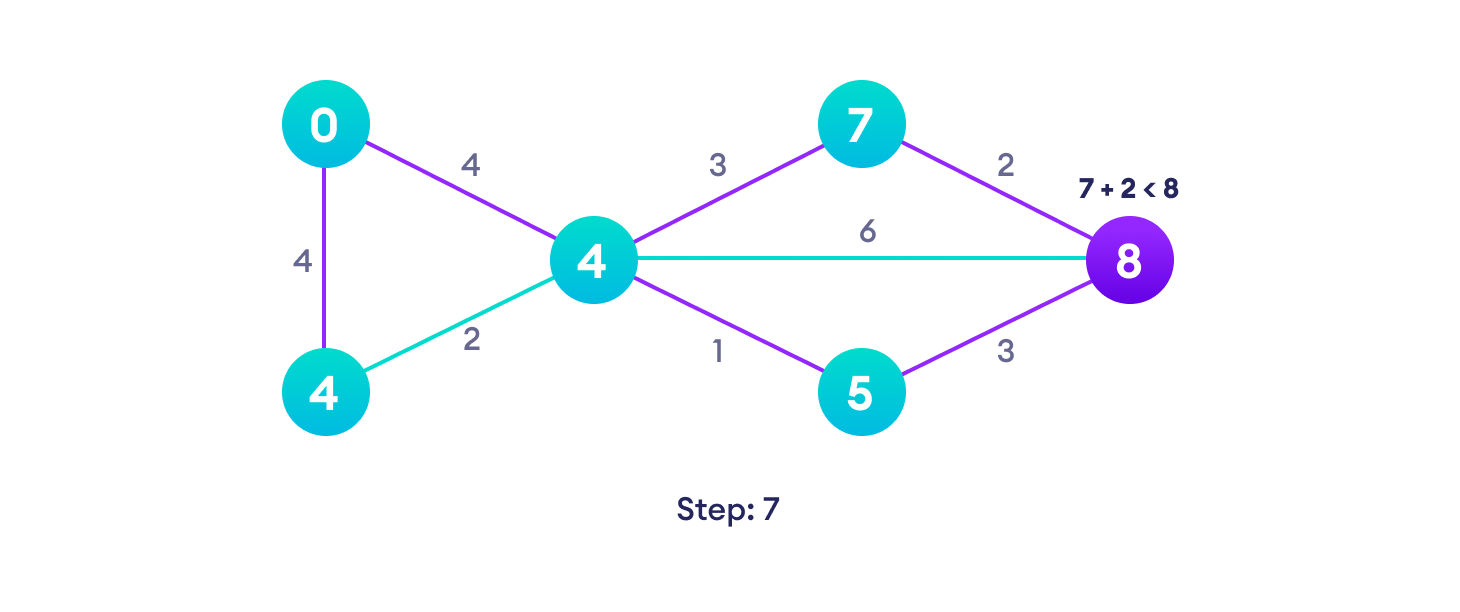Notice how the rightmost vertex has its path length updated twice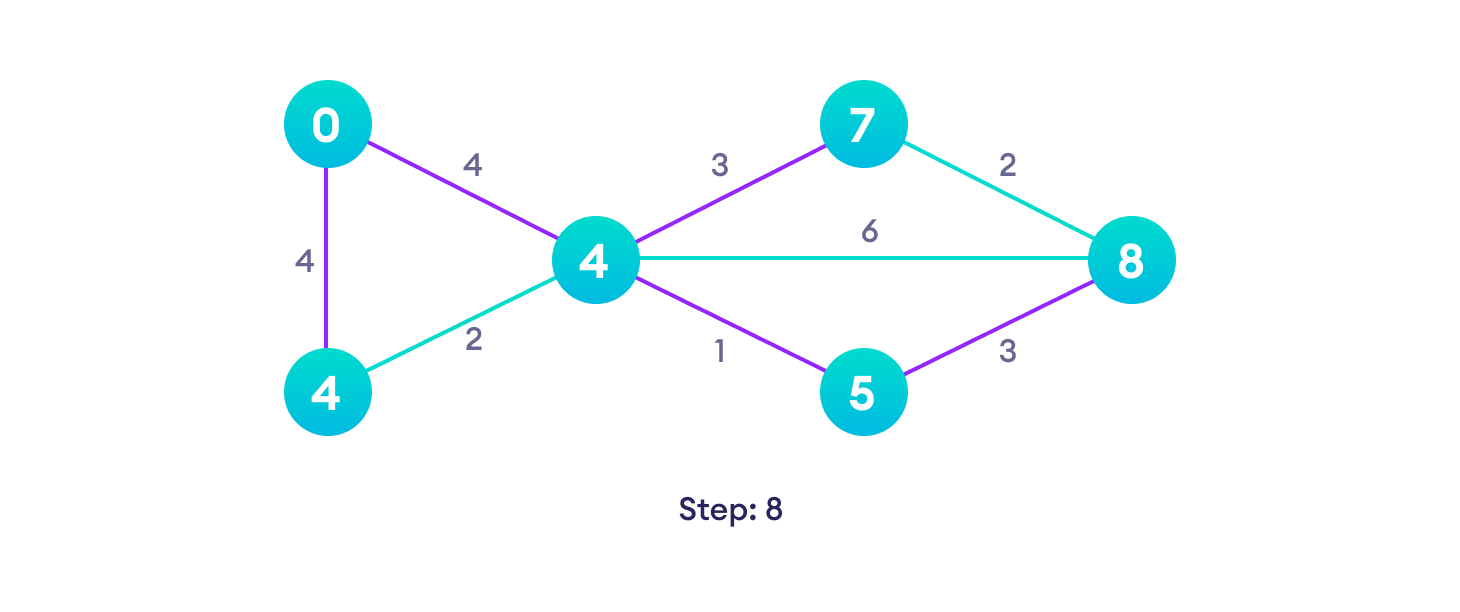Repeat until all the vertices have been visited

## Djikstra’s algorithm pseudocode

We need to maintain the path distance of every vertex. We can store that in an array of size v, where v is the number of vertices.

We also want to be able to get the shortest path, not only know the length of the shortest path. For this, we map each vertex to the vertex that last updated its path length.

Once the algorithm is over, we can backtrack from the destination vertex to the source vertex to find the path.

A minimum priority queue can be used to efficiently receive the vertex with least path distance.

```// Pseudocode
function dijkstra(G, S)
for each vertex V in G
distance[V] <- infinite
previous[V] <- NULL
If V != S, add V to Priority Queue Q
distance[S] <- 0

while Q IS NOT EMPTY
U <- Extract MIN from Q
for each unvisited neighbour V of U
tempDistance <- distance[U] + edge_weight(U, V)
if tempDistance < distance[V]
distance[V] <- tempDistance
previous[V] <- U
return distance[], previous[]```

## Code for Dijkstra’s Algorithm

The implementation of Dijkstra’s Algorithm in C++ is given below. The complexity of the code can be improved, but the abstractions are convenient to relate the code with the algorithm.PythonJavaCC++

```# Dijkstra's Algorithm in Python

import sys

# Providing the graph
vertices = [[0, 0, 1, 1, 0, 0, 0],
[0, 0, 1, 0, 0, 1, 0],
[1, 1, 0, 1, 1, 0, 0],
[1, 0, 1, 0, 0, 0, 1],
[0, 0, 1, 0, 0, 1, 0],
[0, 1, 0, 0, 1, 0, 1],
[0, 0, 0, 1, 0, 1, 0]]

edges = [[0, 0, 1, 2, 0, 0, 0],
[0, 0, 2, 0, 0, 3, 0],
[1, 2, 0, 1, 3, 0, 0],
[2, 0, 1, 0, 0, 0, 1],
[0, 0, 3, 0, 0, 2, 0],
[0, 3, 0, 0, 2, 0, 1],
[0, 0, 0, 1, 0, 1, 0]]

# Find which vertex is to be visited next
def to_be_visited():
global visited_and_distance
v = -10
for index in range(num_of_vertices):
if visited_and_distance[index] == 0 \
and (v < 0 or visited_and_distance[index] <=
visited_and_distance[v]):
v = index
return v

num_of_vertices = len(vertices)

visited_and_distance = [[0, 0]]
for i in range(num_of_vertices-1):
visited_and_distance.append([0, sys.maxsize])

for vertex in range(num_of_vertices):

# Find next vertex to be visited
to_visit = to_be_visited()
for neighbor_index in range(num_of_vertices):

# Updating new distances
if vertices[to_visit][neighbor_index] == 1 and \
visited_and_distance[neighbor_index] == 0:
new_distance = visited_and_distance[to_visit] \
+ edges[to_visit][neighbor_index]
if visited_and_distance[neighbor_index] > new_distance:
visited_and_distance[neighbor_index] = new_distance

visited_and_distance[to_visit] = 1

i = 0

# Printing the distance
for distance in visited_and_distance:
print("Distance of ", chr(ord('a') + i),
" from source vertex: ", distance)
i = i + 1```

## Dijkstra’s Algorithm Complexity

Time Complexity: `O(E Log V)`

where, E is the number of edges and V is the number of vertices.

Space Complexity: `O(V)`

## Dijkstra’s Algorithm Applications

• To find the shortest path
• In social networking applications
• In a telephone network
• To find the locations in the map
045
Previous Post

Next article